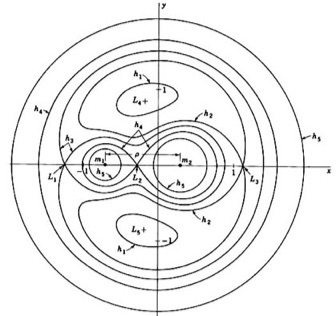# Mon bloc note

## Why has the three-body problem not been solved?

##### 7 Septembre 2015   (619 mots)Un petit post en anglais, issu d’une de mes contributions sur le site Quora

On Quora, the original question was :

Why has the three-body problem not been solved? The two body problem has been completely solved. What are the technical obstacles in solving the three-body problem. Are these difficulties theoritical or not?

TL;DR

It is a little more complicated than what people usually know about it. Solutions have been found, but they are not very useful.

### Longer story

I asked myself the same question, while reading “The Three-Body Problem”, a very good Science-Fiction novel by Liu Cixin.

The issue can be summarized as: how to predict the motion of 3 bodies interacting with each other through gravitation? The problem had been solved since Newton for 2 bodies : the gravitation law allowed one to find the position vectors $$\vec{r}_1(t)$$ and $$\vec{r}_2(t)$$ as simple functions with only $$t$$ (time) as a parameter, given initial conditions of course.

But the N-bodies (with N >2) problem happens to be much more complicated.

Lots of work had been done from the XVIIIth century on, by very famous mathematicians and physicians (Euler, Lagrange, Jacobi, Hill…). Several solutions for particular cases had been solved as early as in 1765.

But until the beginning of the XXth century, no general solution was found. In 1885, a competiton was launched by the King Oscar II from Sweden, in order to find a general solution for the problem, showing a great interest at that time about the problem. Poincaré was awarded that prize for the well known result about non-integrability of the system of equations for the three-body problem.

### Poincaré result

Poincaré, as stated before, showed that one cannot solve the Three-Body Problem through the usual integration method. It is quite simple to understand why.

In a 3-body problem, we have 9 different spatial coordinate components ($$(x_1, y_1, z_1), (x_2, y_2, z_2), (x_3, y_3, z_3)$$) and 9 different velocity components, so we have to solve a system of equations with 18 degrees of freedom.

After some calculation, the gravitional law yields 6 different equations (integrals) of motion, the angular momentum conservation law yields 6 more integrals of motion, the energy conservation law yields 1 more, for a sum of 10 integrals of motion and 18 degrees of freedom.

One finds that there two more integrals of motion (for a total of 12), but Poincaré and Bruns proved that no other independant integrals of motion exist.

That showed that the problem has no usual solution from second order differential equations, and simple $$\vec{r}_i(t)$$ functions.

### Sundman solutions

But that does not mean that there exists no exact solution for the 3-body problem.

After Poincaré’s finding, people started to look for other ways to study the problem. Painlevé conjonctured that power series solutions could prove helpful, and Sundman was the first to derive exact solutions for the 3-body problem.

Sundman was sucessful in finding exact solutions in the form:

$$f(z) = \sum\limits_{k=0}^{\infty}A_k z^{k}$$

However, despite their beauty, it happens that these solutions converge very slowly: “it requires millions of terms to find the motion of one body for insignificantly short durations of time” 

### Summary

It has been proven that there are not “usual” (integral) solutions for the problem. Sundman found exact solutions, but those are not very useful.

So lots of research has been done during the XXth century to find more useful numerical solutions. Physicists have discovered interesting and practicable solutions, but research is still going on to find “better” solutions.

1. Henkel, M., 2002 : Sur la solution de Sundman du probleme des trois corps, arXiv:physics/0203001 [physics.hist-ph]
2. Musielak, Z.E., 2015 : The three-body problem, arXiv:1508.02312 [astro-ph.EP